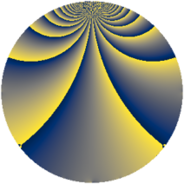# Properties

 Label 169.2.kLevel $169$ Weight $2$ Character orbit 169.k Rep. character $\chi_{169}(4,\cdot)$ Character field $\Q(\zeta_{78})$ Dimension $360$ Newform subspaces $1$ Sturm bound $30$ Trace bound $0$

# Related objects

## Defining parameters

 Level: $$N$$ $$=$$ $$169 = 13^{2}$$ Weight: $$k$$ $$=$$ $$2$$ Character orbit: $$[\chi]$$ $$=$$ 169.k (of order $$78$$ and degree $$24$$) Character conductor: $$\operatorname{cond}(\chi)$$ $$=$$ $$169$$ Character field: $$\Q(\zeta_{78})$$ Newform subspaces: $$1$$ Sturm bound: $$30$$ Trace bound: $$0$$

## Dimensions

The following table gives the dimensions of various subspaces of $$M_{2}(169, [\chi])$$.

Total New Old
Modular forms 408 408 0
Cusp forms 360 360 0
Eisenstein series 48 48 0

## Trace form

 $$360q - 23q^{2} - 24q^{3} - 41q^{4} - 26q^{5} - 32q^{6} - 26q^{7} - 26q^{8} - 11q^{9} + O(q^{10})$$ $$360q - 23q^{2} - 24q^{3} - 41q^{4} - 26q^{5} - 32q^{6} - 26q^{7} - 26q^{8} - 11q^{9} - 27q^{10} - 26q^{11} - 14q^{12} - 34q^{13} - 30q^{14} - 84q^{15} - 13q^{16} - 31q^{17} + 52q^{18} - 33q^{19} - 29q^{20} - 26q^{21} - 33q^{22} + 57q^{23} + 58q^{24} + 2q^{25} - 29q^{26} - 30q^{27} - 26q^{28} - 19q^{29} + 178q^{30} - 78q^{31} - 30q^{32} - 26q^{33} - 91q^{34} - 18q^{35} - 51q^{36} - 41q^{37} + 25q^{38} + 12q^{39} - 134q^{40} - 17q^{41} + 250q^{42} - 28q^{43} + 42q^{45} + 18q^{46} + 117q^{47} - 57q^{48} - 117q^{49} - 20q^{50} - 59q^{51} + 37q^{52} - 75q^{53} + 118q^{54} + 64q^{55} - 42q^{56} - 104q^{57} - 87q^{58} + 170q^{59} + 78q^{60} - 15q^{61} + 19q^{62} + 39q^{63} + 32q^{64} - 17q^{65} + 73q^{66} + 20q^{67} - 76q^{68} - 11q^{69} + 46q^{71} - 198q^{72} - 26q^{73} + 29q^{74} - 70q^{75} + 58q^{76} + 6q^{77} + 128q^{78} - 54q^{79} - 24q^{80} - 7q^{81} + 81q^{82} + 234q^{83} - 273q^{84} - 74q^{85} + 52q^{86} - 112q^{87} + 256q^{88} - 27q^{89} + 28q^{90} - 78q^{91} - 34q^{92} + 51q^{93} + 28q^{94} - 11q^{95} + 143q^{96} + 40q^{97} - 47q^{98} + O(q^{100})$$

## Decomposition of $$S_{2}^{\mathrm{new}}(169, [\chi])$$ into newform subspaces

Label Dim. $$A$$ Field CM Traces $q$-expansion
$$a_2$$ $$a_3$$ $$a_5$$ $$a_7$$
169.2.k.a $$360$$ $$1.349$$ None $$-23$$ $$-24$$ $$-26$$ $$-26$$